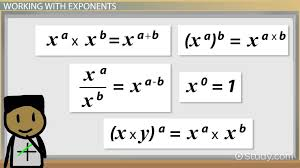# Algebra Formulas: Introduction and Formulas## Algebra Introduction

Algebra is a branch of mathematics that deals with the study of Mathematical symbols and certain rules for operating these symbols. The basic part of algebra is known as elementary algebra whereas the abstract part of algebra is known as abstract algebra or modern algebra. Abstract algebra is useful for any study of Mathematics, Science, and engineering as well as it has applications in medicines and economics. On the other hand, abstract Mathematics is a part of advanced Mathematics which is primarily studied by professional Mathematicians. Algebra formulas are used to find unknown quantities by substituting real-life variables into equations. Algebra formulas include algebraic identities, algebraic equations, roots of quadratic equations, arithmetic progressions, geometric progression, etc.

## List of Algebra Formulas

1. Algebraic Identities

Difference of Squares:   ² – ² = () (  +)

Difference of Cubes: ³ – ³ = () (² + ²+ αβ)

Sum of Cubes: ³ + ³ = () (² + ²αβ)

Algebraic Expressions

( + ) ² = ² + ²+ 2αβ

( –  ) ² = ² + ²2αβ

( + = ³+ ³+ 3αβ² + 3²

( = ³³+ 3αβ² – 3²

The quadratic equation is given in the form of ax² + bx + c = 0. Here a, b, and c are the leading coefficients.

The roots of a quadratic equation are expressed as  x = -b ±b² – 4ac2a

Arithmetic Expression

The arithmetic expression is represented as :  a + (a + d) + (a + 2d) + ( a + 3d)…

Here,

‘a’ is the initial term and ‘d’ is a common difference.

Nth Term

The nth term or Tn of arithmetic depression is given as Tn = a + (n – 1)d

Sum of First N Term

The sum of the first n term of the arithmetic expression is given as Sn = n2[2a + (n-1)d].

Geometric Expression

The geometric expression is represented as a + ar + ar² + ar³

Here,

‘a’ is the initial term and ‘r’ is a common factor.

Nth Term

The nth term or Tn of geometric expression is given as Tn = arn-1

Sum of First N Term

The sum of the first n term of the geometric expression is given as Sn = a( 1 – r)1 – r

The Sum To Infinity

If -1 < r < 1, the sum to infinity, S = a1 – r

# Algebraic Expressions

An algebraic expression is an important concept in Mathematics. Algebraic expressions are defined as numerical expressions that are combined with variables to show how quantities are related to each other.

## How Algebraic Expressions Are Formed?

Algebraic expressions are formed by combining constants and variables. The variable can take several values as it has no fixed value. On the other hand, the constant in the algebraic expression has always a fixed value. The algebraic expression 2a + 5 is formed with the variable “a” first by multiplying the constant 2 by variable “a” and then adding the constant 5 to the product.

## What Are Terms In Algebraic Expressions?

Terms are always added to form algebraic expressions. For example, the expression 2a² + 5 has two terms in it i.e. 2a² and 5. The two terms 2a² and 5 are added to form algebraic expressions (2a² + 5).

## What Are Factors in Algebraic Expression?

As we have in the above expression (2a² + 5), the term 2a² is a product of 2, a, and a. Here, we can say that 2, a, and a are the factors of 2a².

## What Are the Coffiecints of The Term In The Algebraic Expression?

Coefficients are the numerical factors associated with the term. For example, in 2ab, 2 is the coefficient of the term.

When the coefficient of any term is “1”, it is generally omitted. For example, you can simply write “1x” as x.

Education

Education

Education

Education